# python tutorial - Tuple | Tuples in Python - learn python - python programming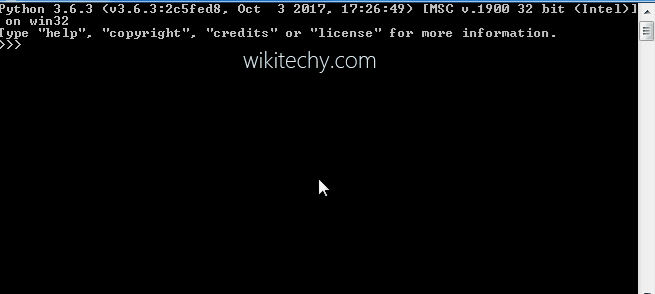Learn Python - Python tutorial - python tuples - Python examples - Python programs

A Tuple is a collection of Python objects separated by commas. In someways a tuple is similar to a list in terms of indexing, nested objects and repetition but a tuple is immutable unlike lists which are mutable.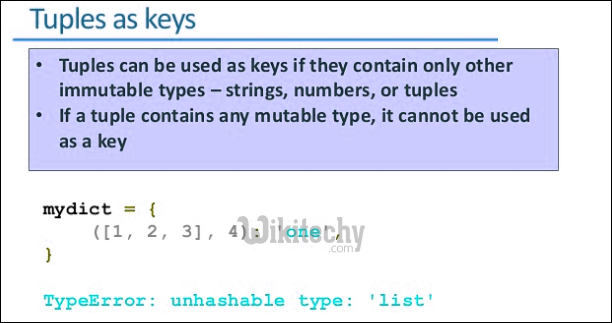Creating Tuples

python tutorial - Output :

python - Sample - python code :

python tutorial - Output :

Note: In case your generating a tuple with a single element, make sure to add a comma after the element.

Concatenation of Tuples

Output:

Nesting of Tuples

python tutorial - Output :

Repetition in Tuples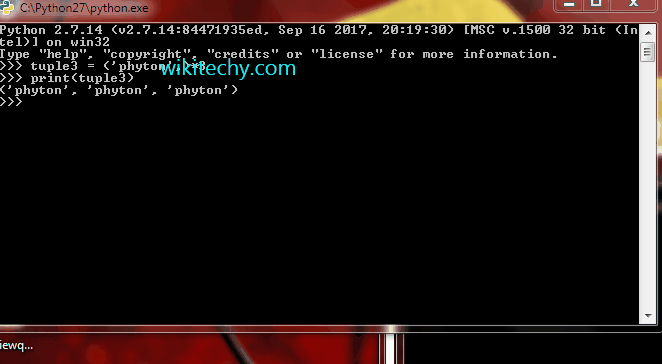Learn Python - Python tutorial - python repetition tuples - Python examples - Python programs

python tutorial - Output :

Try the above without a comma and check. You will get tuple3 as a string ‘pythonpythonpython’.

Immutable Tuples

python tutorial - Output :

Slicing in Tuples

python tutorial - Output :

Deleting a Tuple

Error:

python tutorial - Output :

Finding Length of a Tuple

python tutorial - Output :

Converting list to a Tuple

python tutorial - Output :

Takes a single parameter which may be a list,string,set or even a dictionary( only keys are taken as elements) and converts them to a tuple.

Tuples in a loop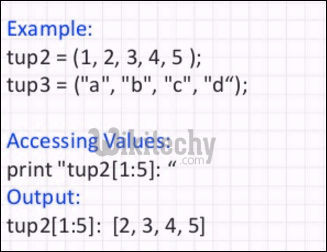python - Sample - python code :

python tutorial - Output :

Using cmp(), max() , min()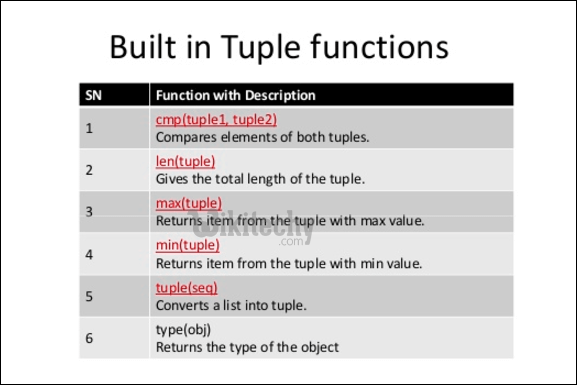python - Sample - python code :

python tutorial - Output :

Note: max() and min() checks the based on ASCII values. If there are two strings in a tuple, then the first different character in the strings are checked.

Wikitechy tutorial site provides you all the learn python , learn to program in python , python coding class , learn python programming language online , learn python coding , learn how to code python , free python course online , python training online free , python book , python developer , python online course free